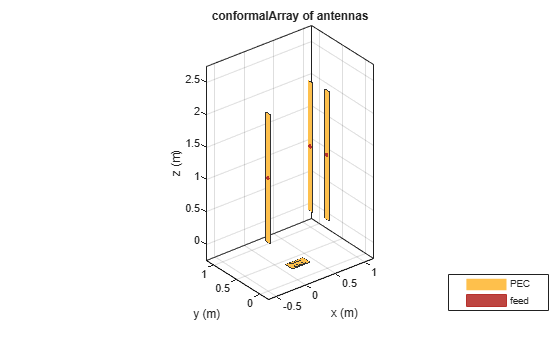# conformalArray

Create conformal antenna array

## Description

The `conformalArray` class creates an antenna array using any element from the antenna or array library. You can also specify an array of any arbitrary geometry, such as a circular array, a nonplanar array, an array with nonuniform geometry, or a conformal array of arrays.

Conformal arrays are used in:

• Direction-finding systems that use circular arrays or stacked circular arrays

• Aircraft systems due to surface irregularities or mechanical stress## Creation

### Syntax

``array = conformalArray``
``array = conformalArray(Name,Value)``

### Description

example

``` `array = conformalArray` creates a conformal antenna array using the default antenna element, shape, and antenna positions. ```

example

``` `array = conformalArray(Name,Value)` creates a conformal antenna array with additional properties specified by one or more name-value pair arguments. `Name` is the property name and `Value` is the corresponding value. You can specify several name-value pair arguments in any order as `Name1`, `Value1`,``` ...```, `NameN`, `ValueN`. Properties not specified retain default values.```

## Properties

expand all

Position of the feed or origin for each antenna element, specified as an M-by-3 real matrix. M is the number of element positions. By default, M is `2`. To specify additional antenna elements, add additional element positions in the conformal array.

Example: `'ElementPosition'`,```[0.1 0.1 0.1; -0.1 -0.1 -0.1;0.2 0.2]```

Data Types: `double`

Individual antenna or array elements in the array, specified as one of the following values:

• A scalar

• An array of objects

• A cell array of objects

By default, a conformal array has two antenna elements, the dipole and the bowtie. To specify additional antenna or array elements, add additional element positions in the conformal array. You can add both balanced and unbalanced antennas to the same conformal array.

Example: `m = monopole`; ```h = conformalArray('Element', [m,m])```. Creates a conformal array consisting of two monopoles antenna elements.

Example: `la = linearArray`; ```ra = rectangularArray```; ```h = conformalArray('Element', {la,ra})```. Creates a conformal array consisting of a linear array and a rectangular array.

Data Types: `cell`

Position reference for the antenna element, specified as either `'origin'` or `'feed'`. For more information, see Position Reference.

Example: `'Reference'`,`'origin'`

Data Types: `char` | `string`

Excitation amplitude of the antenna elements, specified as a scalar or a nonnegative vector. To model dead elements, set the property value to `0`.

Example: `'AmplitudeTaper',3`

Example: `'AmplitudeTaper',[3 0]`. Creates a two-element conformal array, where `3` and `0` are the excitations amplitudes of two elements.

Data Types: `double`

Phase shift for antenna elements, specified as a scalar or a real vector in degrees.

Example: `'PhaseShift',[-45 -45 45 45]`

Data Types: `double`

Tilt angle of the array specified as a scalar or vector with each element unit in degrees. For more information, see Rotate Antennas and Arrays.

Example: `'Tilt',90`,

Example: `'Tilt',[90 90]`,`'TiltAxis',[0 1 0;0 1 1]` tilts the array at 90 degrees about the two axes, defined by vectors.

Data Types: `double`

Tilt axis of the array, specified as:

• Three-element vectors of Cartesian coordinates in meters. In this case, each vector starts at the origin and lies along the specified points on the X-, Y-, and Z-axes.

• Two points in space, each specified as three-element vectors of Cartesian coordinates. In this case, the array rotates around the line joining the two points in space.

• A string input describing simple rotations around one of the principal axes, 'X', 'Y', or 'Z'.

Example: `'TiltAxis',[0 1 0]`

Example: `'TiltAxis',[0 0 0;0 1 0]`

Example: `array.TiltAxis = 'Z'`

Data Types: `double`

## Object Functions

 `show` Display antenna or array structure; display shape as filled patch `info` Display information about antenna or array `beamwidth` Beamwidth of antenna `charge` Charge distribution on metal or dielectric antenna or array surface `correlation` Correlation coefficient between two antennas in array `current` Current distribution on metal or dielectric antenna or array surface `design` Design prototype antenna or arrays for resonance at specified frequency `EHfields` Electric and magnetic fields of antennas; Embedded electric and magnetic fields of antenna element in arrays `impedance` Input impedance of antenna; scan impedance of array `layout` Display array or PCB stack layout `mesh` Mesh properties of metal or dielectric antenna or array structure `optimize` Optimize antenna or array using SADEA optimizer `pattern` Radiation pattern and phase of antenna or array; Embedded pattern of antenna element in array `patternAzimuth` Azimuth pattern of antenna or array `patternElevation` Elevation pattern of antenna or array `returnLoss` Return loss of antenna; scan return loss of array `sparameters` S-parameter object

## Examples

collapse all

Create a default conformal array.

`c = conformalArray`
```c = conformalArray with properties: Element: {[1x1 dipole] [1x1 bowtieTriangular]} ElementPosition: [2x3 double] Reference: 'feed' AmplitudeTaper: 1 PhaseShift: 0 Tilt: 0 TiltAxis: [1 0 0] ```
`show(c)`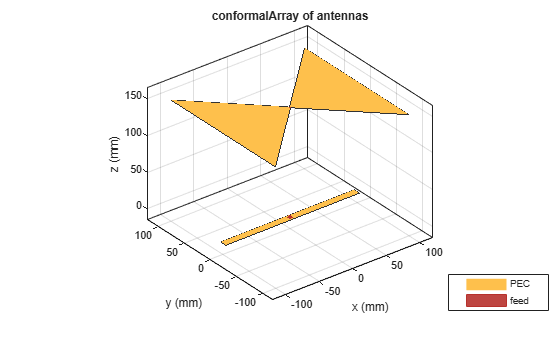Define the radius and the number of elements for the array.

```r = 2; N = 12;```

Create an array of 12 dipoles.

`elem = repmat(dipole('Length',1.5),1,N);`

Define the x,y,z values for the element positions in the array.

```del_th = 360/N; th = del_th:del_th:360; x = r.*cosd(th); y = r.*sind(th); z = ones(1,N); pos = [x;y;z];```

Create a circular array using the defined dipoles and then visualize it. Display the layout of the array.

```c = conformalArray('Element',elem,'ElementPosition',pos'); show(c)```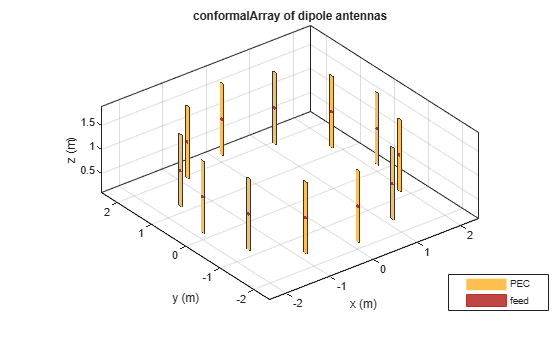```figure layout(c)```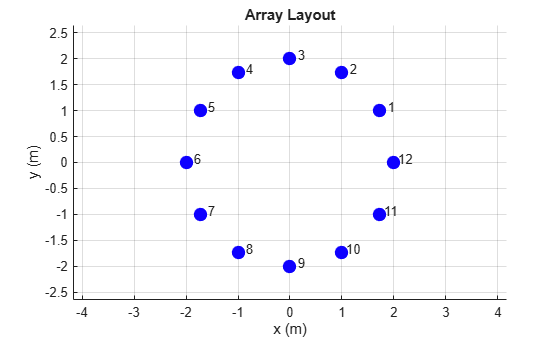Change the width of the fourth and the twelfth element of the circular array. Visualize the new arrangement.

```c.Element(4).Width = 0.05; c.Element(12).Width = 0.2; figure show(c)```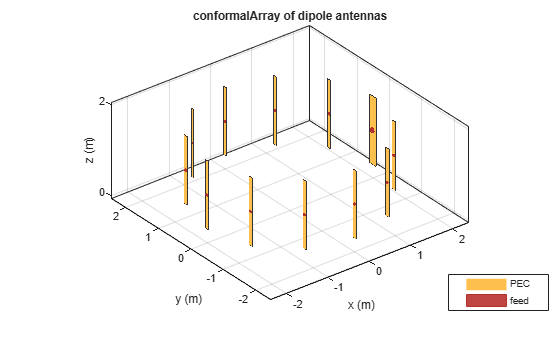Calculate and plot the impedance of the circular array at 100 MHz. The plot shows the impedance of the first element in the array.

```figure impedance(c,100e6)```To view the impedance of all the elements in the array change the value from 1 to 1:12 as shown in the figure.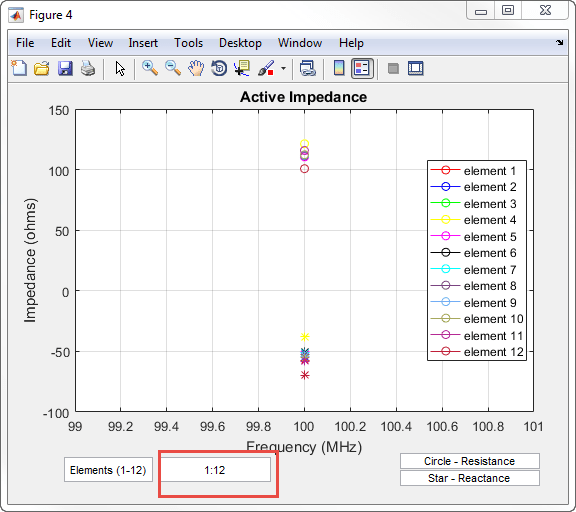Define three circular loop antennas of radii 0.6366 m (default), 0.85 m, and 1 m, respectively.

```l1 = loopCircular; l2 = loopCircular('Radius',0.85); l3 = loopCircular('Radius',1);```

Create a concentric array that uses the origin of circular loop antennas as its position reference.

```c = conformalArray('Element',{l1,l2,l3},'ElementPosition',[0 0 0;0 0 0;... 0 0 0],'Reference','origin'); show(c)```Visualize the radiation pattern of the array at 80 MHz.

`pattern(c,80e6)`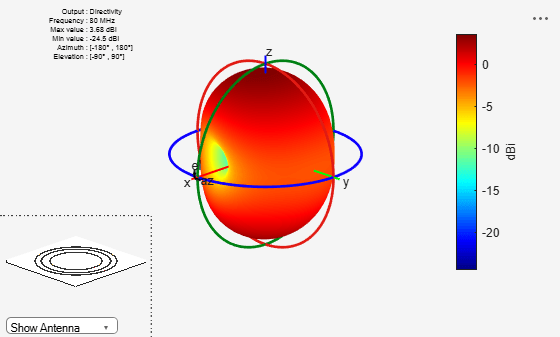Create a dipole antenna to use in the reflector and the conformal array.

`d = dipole('Length',0.13,'Width',5e-3,'Tilt',90,'TiltAxis','Y');`

Create an infinite groundplane reflector antenna using the dipole as exciter.

`rf = reflector('Exciter',d,'Spacing',0.15/2,'GroundPlaneLength',inf);`

Create a conformal array using 36 dipole antennas and one infinite groundplane reflector antenna. View the array.

```x = linspace(-0.4,0.4,6); y = linspace(-0.4,0.4,6); [X,Y] = meshgrid(x,y); pos = [X(:) Y(:) 0.15*ones(numel(X),1)]; for i = 1:36 element{i} = d; end element{37} = rf; lwa = conformalArray('Element',element,'ElementPosition',[pos;0 0 0.15/2]); show(lwa)```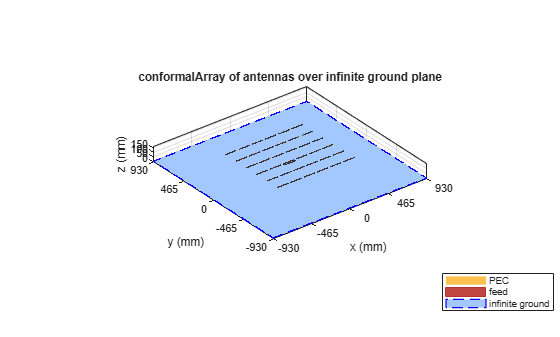Drive only the reflector antenna with an amplitude of 1.

```V = zeros(1,37); V(end) = 1; lwa.AmplitudeTaper = V;```

Compute the radiation pattern of the conformal array.

```figure pattern(lwa,1e9,'Type','efield')```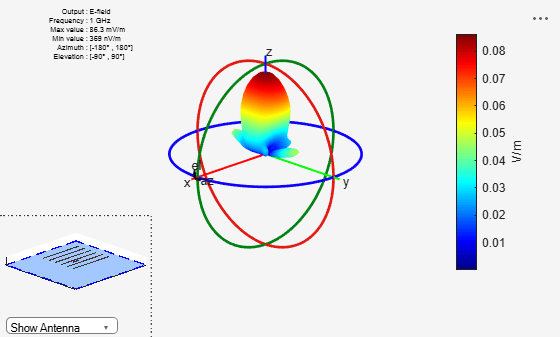Create two patch microstrip antennas using dielectric substrate `FR4`. Tilt the second patch microstrip antenna by 180 degrees.

```d = dielectric('FR4'); p1 = patchMicrostrip('Substrate',d); p2 = patchMicrostrip('Substrate',d,'Tilt',180);```

Create and view a conformal array using the two patch microstrip antennas placed 11 cm apart.

`c = conformalArray('ElementPosition',[0 0 0;0 0 0.1100],'Element',{p1,p2})`
```c = conformalArray with properties: Element: {[1x1 patchMicrostrip] [1x1 patchMicrostrip]} ElementPosition: [2x3 double] Reference: 'feed' AmplitudeTaper: 1 PhaseShift: 0 Tilt: 0 TiltAxis: [1 0 0] ```
`show(c)`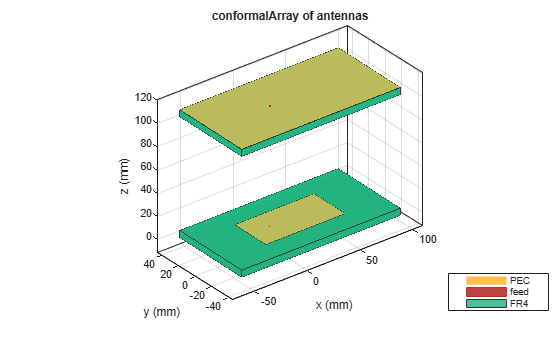Create a conformal array using dipole and monopole antennas.

`c = conformalArray('Element', {dipole, monopole})`
```c = conformalArray with properties: Element: {[1x1 dipole] [1x1 monopole]} ElementPosition: [2x3 double] Reference: 'feed' AmplitudeTaper: 1 PhaseShift: 0 Tilt: 0 TiltAxis: [1 0 0] ```
`c.ElementPosition = [0 0 0; 1.5 0 0];`

Visualize the array.

```figure; show(c);```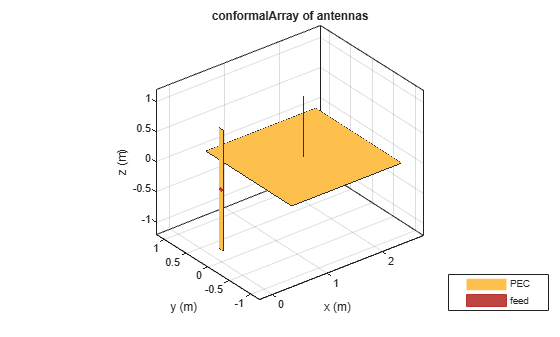Plot the radiation pattern of the array at 70 MHz.

`pattern(c, 70e6)`Create a subarray of linear arrays at different locations.

`la = linearArray('ElementSpacing',1)`
```la = linearArray with properties: Element: [1x1 dipole] NumElements: 2 ElementSpacing: 1 AmplitudeTaper: 1 PhaseShift: 0 Tilt: 0 TiltAxis: [1 0 0] ```
`subArr = conformalArray('Element',[la la],'ElementPosition',[1 0 0;-1 1 0])`
```subArr = conformalArray with properties: Element: [1x2 linearArray] ElementPosition: [2x3 double] Reference: 'feed' AmplitudeTaper: 1 PhaseShift: 0 Tilt: 0 TiltAxis: [1 0 0] ```
`show(subArr)`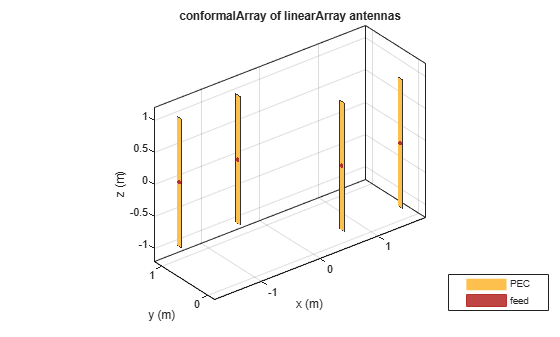Create a linear array of dipoles with and element spacing of 1m.

`la = linearArray('ElementSpacing',1);`

Create a rectangular array of microstrip patch antennas.

`ra = rectangularArray('Element',patchMicrostrip,'RowSpacing',0.1,'ColumnSpacing',0.1);`

Create a subarray containing the above linear and rectangular arrays with changes in amplitude taper and phase shift values.

```subArr = conformalArray('Element',{la ra dipole},'ElementPosition',[0 0 1.5;0 0 0;1 1 1],... 'AmplitudeTaper',[3 0.3 0.03],'PhaseShift',[90 180 120]); show(subArr)```